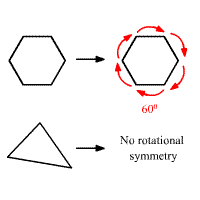# Rotational Symmetry

A figure has rotational symmetry if it can be rotated by an angle between $0°$ and $360°$ so that the image coincides with the preimage.

The angle of rotational symmetry is the smallest angle for which the figure can be rotated to coincide with itself.

The order of symmetry is the number of times the figure coincides with itself as its rotates through $360°$ .

Example:

A regular hexagon has rotational symmetry. The angle of rotation is $60°\text{\hspace{0.17em}}$ and the order of the rotational symmetry is $6$ .

A scalene triangle does not have rotational symmetry.